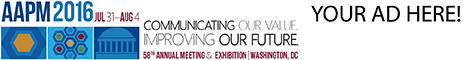# Program Information

## Diffusion Synthetic Acceleration for Linear Boltzmann Transport EquationG Chen

## Presentations

#### SU-E-T-270 (Sunday, July 12, 2015) 3:00 PM - 6:00 PM Room: Exhibit Hall

Purpose: Linear Boltzmann transport equation (LBTE) is as accurate as the Monte Carlo method (MC) for dose calculation in photon/particle therapy (LBTE is a deterministic and Eulerian formulation and MC is a statistical and Lagrangian description). An advantage of LBTE is that numerous acceleration techniques can be utilized for acceleration. This work is to explore the acceleration of LBTE via diffusion synthetic acceleration (DSA).

Methods: For simplicity, two-dimensional, steady-state, and within-group LBTE is considered with two angular dimensions and two spatial dimensions. The discrete ordinate method is developed for solving this integro-differential equation. The angular variables are discretized using a level-symmetric quadrature set on the unit sphere. The spatial variables are discretized on the structured grid based on the diamond scheme. The source-iteration method (SI) is used to solve the discretized system.
Since SI is slow in optically thick and highly scattering regime. DSA is developed to accelerate SI. The motivation for DSA is that diffusion equation (DE) is a good approximation of LBTE in the above regime. However, DE is much cheaper than LBTE computationally since DE only involves spatial variables. Thus, in each DSA iteration, DSA adds to the SI step a computationally-negligible DE step, i.e., to first solve DE with the SI residual as source term, and then compensate the SI solution with DE solution.

Results: DSA was benchmarked and compared with SI. The difference between two methods was within 0.12% which verifies the accuracy of DSA, while DSA demonstrated the great advantage in speed, e.g., the reduction of iteration number to 6% and 4% respectively for cases with 100 and 1,000 scattering-absorption ratio that commonly occur in clinical dose calculation.

Conclusion: DSA has been developed as one of many possible means for accelerating the numerical solver of LBTE for dose calculation.

Funding Support, Disclosures, and Conflict of Interest: The authors were partially supported by the NSFC (#11405105), the 973 Program (#2015CB856000) and the Shanghai Pujiang Talent Program (#14PJ1404500).

Contact Email: